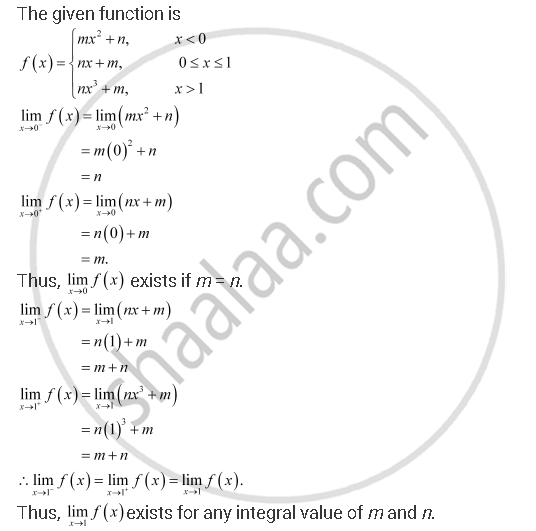CBSE (Commerce) Class 11CBSE
Share
Notifications

View all notifications
Books Shortlist
Your shortlist is empty

# Solution for For What Integers M and N Does Limit (X-> 0) F(X) and Limit (X -> 1) F(X) Exist? - CBSE (Commerce) Class 11 - Mathematics

Login
Create free account

Forgot password?
ConceptLimits Limits of Polynomials and Rational Functions

#### Question

if f(x) = { (mx^2 + n, x < 0),(nx + m, 0<= x <= 1),(nm^3 + m, x > 1):}

For what integers m and n does lim_(x-> 0) f(x) and lim_(x -> 1) f(x) exist?

#### SolutionIs there an error in this question or solution?

#### APPEARS IN

NCERT Solution for Mathematics Textbook for Class 11 (2013 to Current)
Chapter 13: Limits and Derivatives
Q: 32 | Page no. 303
Solution For What Integers M and N Does Limit (X-> 0) F(X) and Limit (X -> 1) F(X) Exist? Concept: Limits - Limits of Polynomials and Rational Functions.
S